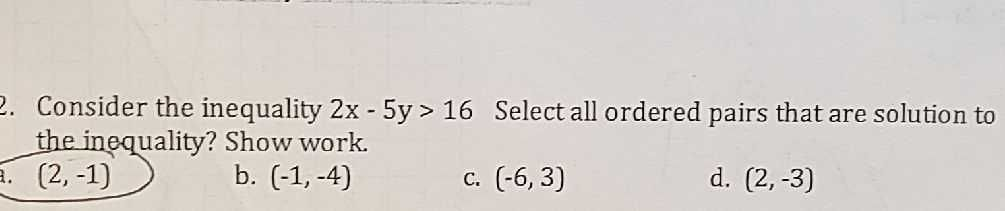### ¿Todavía tienes preguntas de matemáticas?

Pregunte a nuestros tutores expertos
Algebra
Pregunta2. Consider the inequality $$2 x - 5 y > 16$$ Select all ordered pairs that are solution to the inequality? Show work. a. $$( 2 , - 1 )$$ b. $$( - 1 , - 4 )$$ c. $$( 2 , - 3 )$$ d. $$( 2 , - 3 )$$